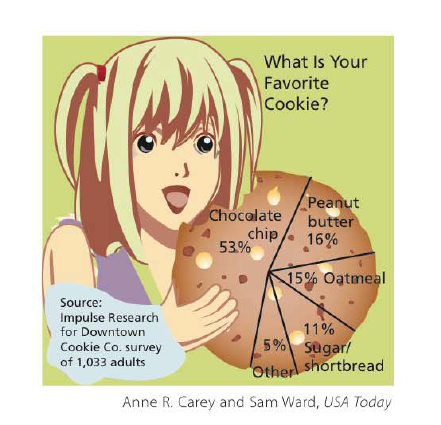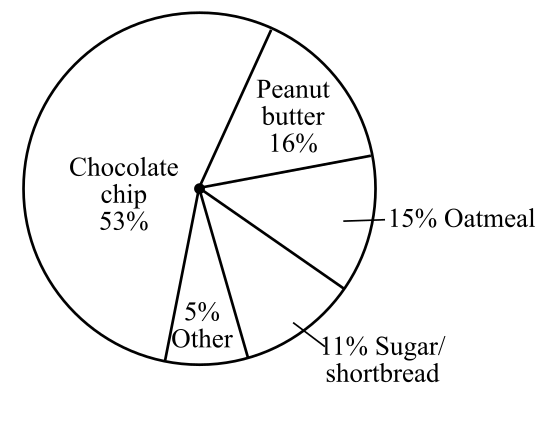Chapter 6.I, Problem 43RE### Contemporary Mathematics for Busin...

8th Edition
Robert Brechner + 1 other
ISBN: 9781305585447

#### Solutions

Chapter
Section### Contemporary Mathematics for Busin...

8th Edition
Robert Brechner + 1 other
ISBN: 9781305585447
Textbook Problem

# Use the pie chart “What is Your Favorite Cookie?” to find the decimal and reduced fraction equivalent for exercises 41–45. Type of Cookie Decimal Reduced Fraction 43. OatmealTo determine

To calculate: The decimal and reduced fraction value of the oatmeal cookies by using the pie chart.Explanation

Given Information:

The provided pie chart is,

Formula used:

Follow the steps as mentioned below to convert percentage value into decimal value.

Step1: Take away the percentage sign.

Step2: Divide the value obtained in step 1 by 100.

Follow the steps as mentioned below to convert percentage value into reduced fraction value.

Step1: Take away the percentage sign.

Step2: Now put the number with the value of percent as the numerator and 100 as the denominator, then reduce the fraction to its lowest term.

Calculation:

From the provided pie chart the value of oatmeal cookies is 15%.

Compute the decimal value of oatmeal cookies as follows:

Take away the percentage sign,

15

Divide by 100 and simplify,

15100=0

### Still sussing out bartleby?

Check out a sample textbook solution.

See a sample solution

#### The Solution to Your Study Problems

Bartleby provides explanations to thousands of textbook problems written by our experts, many with advanced degrees!

Get Started

#### (13)0

Applied Calculus for the Managerial, Life, and Social Sciences: A Brief Approach

#### In problems 45-62, perform the indicated operations and simplify. 50.

Mathematical Applications for the Management, Life, and Social Sciences

#### Using for |x| < 1 and differentiation, find a power series for .

Study Guide for Stewart's Single Variable Calculus: Early Transcendentals, 8th